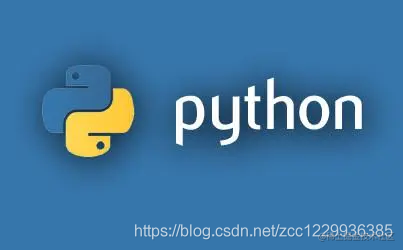# 【不会吧不会吧，不会有人真的三分钟入门Python了吧？】Python编程基础# python编程基础

## 变量

### 字符串

``````string = 'hello world.'
name = "cczhu"
print(string) #hello world.
print(name) #cczhu

``````hello world.
cczhu

### 数字

Python数字数据类型用于存储数值。常用的类型包括整型（int）、浮点型（float）

``````age = 18
average = 222.222

print(age) #18
print(average) #222.222

``````18
222.222

### 列表

``````books = ['book1', 'book2', 'book3']
data = ['cczhu', 27, books]

print(books) #book1
print(data[-1]) #['book1', 'book2', 'book3']
print(data) #['cczhu', 27, ['book1', 'book2', 'book3']]
print(data) #book1

``````book1
['book1', 'book2', 'book3']
['cczhu', 27, ['book1', 'book2', 'book3']]
book2

### 元组

``````tuple_1 = ("strng", 1, ["a", "b" , "c"], 4)
tuple_2 = ("hi",)
empty_tuple = tuple()
print(tuple_1)

``````('strng', 1, ['a', 'b', 'c'], 4)

### 字典

``````dic_1 = {
'name':'cczhu',
"age":'22',
'books':['book1', 'book2', 'book3']
}

dic_2 = dict(
name='cczhu',
age=22,
books=['book1', 'book2', 'book3']
)

print(dic_1)
print(dic_1['name'])
print(dic_1['books'])

``````{'age': '22', 'books': ['book1', 'book2', 'book3'], 'name': 'cczhu'}
cczhu
book2

### 集合

``````my_list = ['book1', 'book2', 'book2', 'book4']
my_test = {'book1', 'book2', 'book2', 'book4'}
your_test = {'book1', 'book2', 'book3'}

print(my_list)
print(set(my_list))
print(my_test)

print(my_test - your_test)
print(my_test | your_test)
print(my_test & your_test)
print(my_test ^ your_test)

``````['book1', 'book2', 'book2', 'book4']
set(['book1', 'book2', 'book4'])
set(['book1', 'book2', 'book4'])
set(['book4'])
set(['book1', 'book2', 'book3', 'book4'])
set(['book1', 'book2'])
set(['book3', 'book4'])

## 运算符

### 算术运算符

+加，x加ya+b 输出结果30
-减，x减ya-b 输出结果-10
*乘，两个数相乘或返回一个被重复若干次的字符串a*b输出结果200
/除，x除以yb/a输出结果2
%取模，返回除法的余数b%a输出结果0
**幂，返回x的y次幂a**b为10的20次方，输出结果100,000,000,000,000,000,000
//取整除，返回商的整数部分9//2输出结果4,9.0//2.0输出结果4.0

### 比较运算符

==等于，比较对象是否相等(a == b)返回False
!=不等于，比较对象是否不相等(a != b)返回True
<>不等于，比较对象是否不相等(a <> b) 返回True
>大于，返回x是否大于y(a > b) 返回False
<小于，返回x是否小于y(a < b)返回True
>=大于等于，返回x是否大于等于y(a >= b) 返回False
<=小于等于，返回x是否小于等于y(a <= b)返回True

### 赋值运算符

=简单的赋值运算符c=a+b 将a+b的运算结果赋值给c
+=加法赋值运算符a+=b 等价于a = a + b
-=减法赋值运算符a-=b 等价于a = a -b
*=乘法赋值运算符a*=b等价于a = a * b
/=除法赋值运算符a/=b等价于a = a / b
%=取模赋值运算符a%=b等价于a = a %b
**=幂赋值运算符a **= b 等价于a = a ** b
//=取整数赋值运算符a//=b等价于a = a //b

### 逻辑运算符

and与关系，如果x为False，x and y返回x的值，否者返回y的计算值(a and b) 返回20
or或关系，如果x是True,它返回x的值，否则它返回y的计算值(a or b) 返回10
not如果x为True，返回False。如果x为False，它返回True。not(a and b) 返回False

### 成员运算符

is两个变量引用自同一个对象则返回Truex is y，类似id(x) == id(y)，如果引用的是同一个对象则返回True，否者返回False
is not两个变量不是引用自同一个对象返回Truex is not y ,类似于id（a）= !(b)。如果应用的不是同一个对象则结果返回正确接人True，否者返回False

### 身份运算符

is两个变量引用自同一个对象则返回Truex is y，类似id(x) == id(y),如果引用的是同一个对象则返回True，否则返回False
is not两个变量不是引用自同一个对象返回Truex is not y，类似id(a) != id(b)。如果引用的不是同一个对象则返回结果True，否则返回False

### 位运算符

&与运算（两个同时为1，结果为1，否则为0）
|或运算（一个为1，其值为1，否则为0）
^异或运算（值不同，则该位结果为1，否则为0）
~取反运算（对数据的每个二进制位取反，即把1变为0，把0变为1。~a类似-a-1）
<<左移运算（运算数的各二进制位全部左移若干位，由“<<”右边的数指定移动的位数，高位丢弃，低位补0）
>>右移运算（把“>>”左边的运算数的各二进制全部右移若干位，“>>”右边的数指定移动的位数）

## 语句

### 条件循环语句：while

while条件:

codeblock1

codeblock2

.....

while运行步骤：

step1:如果条件为真，那么依次执行：codeblock1、codeblock2、....

step2:再次判断条件，如果为True则再次执行：codeblock1、codeblock2、....，如果条件为False，则循环终止。

#### while+break

break语句可以跳出for和while的循环体

#### while+continue

continue用于结束本次循环，直接进入下次循环。

### 迭代循环语句：for

for循环可以遍历任何可选代对象，如一个列表或者一个字符串。break和continue也可用于for循环，同while循环。

## 函数与类

### 函数

#### 定义一个函数

• 语法
``````def 函数名(参数1, 参数2, 参数3,...);
'''注释'''
函数体
return 返回值

• 使用原则

• 位置参数

• 关键字参数

• 默认参数

• 可变长参数

• _init_方法

• self

### 模块

#### 概念

• import
``````import time #导入时间模块
print(time.time())

• from ... import
``````from time import time #从time模块中只导入一个time方法
print(time())

• from ... import *语句
``````from time import * #将time模块中的所有内容全部导入
print(time())

## python技术体系

### python网络爬虫

requests/beautifulsoup库、scrapy框架

Numpy、Scipy

Pandas

### python数据可视化

Matplotlib、Seaborn、Pyecharts

PySpark、PyHive

### python机器学习

Scikit-Learn、Xgboost

Tensorflow

## 结束寄语

``````写博不易，如蒙厚爱，赏个关注，一键三连~~点赞+评论+收藏🤞🤞🤞，感谢您的支持~~